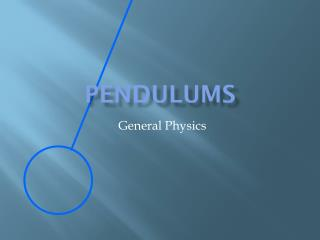DownloadDownload PresentationPendulums

# Pendulums

Download Presentation## Pendulums

- - - - - - - - - - - - - - - - - - - - - - - - - - - E N D - - - - - - - - - - - - - - - - - - - - - - - - - - -
##### Presentation Transcript

1. Pendulums • General Physics

2. Periodic Motion • A motion that is regular and repeating • Examples • Circular motion • Mass bouncing up and down on a spring • Pendulums

3. Simple Harmonic Motion (SHM) • motion that returns an object to its rest position • caused by a restoring force that is directly proportional to the objects displacement

4. Measuring the Motion of a Pendulum • Bob: • the mass that at is hung on the end of a string that makes up the pendulum • measured in kg • Length: • Measured from point of attachment to center of bob in the rest position. • measured in meters

5. Measuring the motion of a pendulum • Rest position: • Also known as the equilibrium position • Located at bottom of swing • Period: • the time for one complete cycle (forward and back to same position) • symbol: T • unit: seconds

6. Measuring the Motion of a Pendulum • Frequency: • The number of complete cycles in a period of time • Symbol: f • Unit: 1/s or Hertz

7. Measuring the Motion of a Pendulum • Amplitude: • The maximum displacement from the rest position. • Symbol: A • Unit: m • We will measure the amplitude of a pendulum by using a protractor to measure the degrees that the bob is pulled back from the rest position.

8. Equation to find Period T=2Π(l/g)1/2 l= length g=acceleration due to gravity

9. Equation to find local acceleration of gravity g = 4Π2(length)/T2